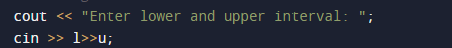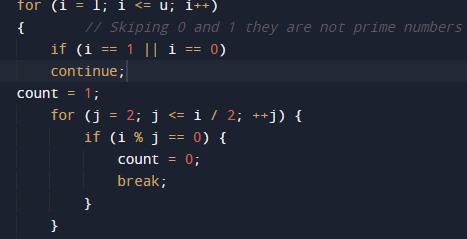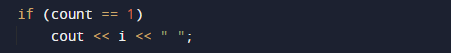# C++ Program to Display Prime Numbers Between Two Numbers

In this tutorial you will learn about the C++ Program to Display Prime Numbers Between Two Numbers and its application with practical example.

In this tutorial, we will learn to create a C++ program that will check that provided number is Prime or Not using C++ programming.

## Prerequisites

Before starting with this tutorial we assume that you are best aware of the following C programming topics:

• Operators.
• Basic input/output.
• Basic C++ Programming.
• If else statement.
• Looping statements.

## What Is Prime number?

Any positive integer number which is greater than 1 (Num > 1 ) and only it can be divisible by itself and by 1 and it has max two factors is called as prime number.Rather we can say that a prime number is a number which only divided by himself or 1.

## Example:->

Note:  2  is the only positive(even) and smallest Prime number.In our program we will find Prime numbers between two numbers using for loop.

## C++ Program to Display Prime Numbers Between Two Numbers

Firstly we declare required header file and variable and also initiates required values in variable. Next we take value from user at run time and then after we will find that the given value is prime number or not.Let’s take a look to the program.

## OutputIn the above program, we have first declared and initialized a set variables required in the program.

• l = it will hold lower value.
• u=it will hold upper limit.
• i and j= for iteration.
• count = count will print prime numbers is count=1.

In the next statement user will enter two number which will be assigned in variables ‘l’ and ‘u’.lower and upper limit of loop Now loop start from l to given number(u).In ever iteration we will check  the numbers between the interval  l and u . For each number with in the for loop, will be checked if this number is prime value of count =1 if not the value of count remain zero(0).If count=1 it is a prime then print the number.Then the next number in the loop is checked, till all numbers  between  l and u are checked.And finally we print all the number between given numbers.In this tutorial we have learn about the C++ Program to Display Prime Numbers Between Two Numbers and its application with practical example. I hope you will like this tutorial.